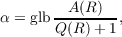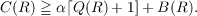#### Vol. 15, No. 2, 1965

 Download this articleFor screen For printingRecent Issues Vol. 325: 1  2 Vol. 324: 1  2 Vol. 323: 1  2 Vol. 322: 1  2 Vol. 321: 1  2 Vol. 320: 1  2 Vol. 319: 1  2 Vol. 318: 1  2Online Archive Volume: Issue:The Journal Subscriptions Editorial Board Officers Contacts Submission Guidelines Submission Form Policies for Authors ISSN: 1945-5844 (e-only) ISSN: 0030-8730 (print) Special Issues Author Index To Appear Other MSP Journals
An inequality for the number of elements in a sum of two sets of lattice points

### Betty Kvarda

Vol. 15 (1965), No. 2, 545–550
##### Abstract

For a fixed positive integer n, let Q be the set of all n-dimensional lattice points (x1,,xn) with each xi a nonnegative integer and at least one xi positive. A finite nonempty subset R of Q is called a fundamental set if for every (r1,,rn) in R, all vectors (x1,,xn) of Q with xi ri, i = 1,,n, are also in R. If A is any subset of Q and R is any fundamental set, let A(R) denote the number of vectors in A R. Finally, if A is any proper subset of Q, let the density of A be the quantitytaken over all fundamental sets R for which A(R) < Q(R). Then the theorem proved in this paper can be stated as follows.

Theorem. Let A and B be subsets of Q, let C be the set of all vectors of the form a, b, or a + b where a A and b B, let α be the density of A, and let R be any fundamental set such that (1) there exists at least one vector in R which is not in C, and (2) for each b in B R (if any) there exists g in R but not in C such that g b is in Q. ThenPrimary: 10.45
##### Milestones